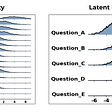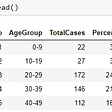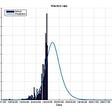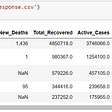# Import Libraries

`import pandas as pdimport numpy as npimport seaborn as snsimport matplotlib.pyplot as plt%matplotlib inlinefrom sklearn.ensemble import RandomForestClassifier, ExtraTreesClassifierfrom sklearn.model_selection import cross_val_score, train_test_split, GridSearchCVfrom sklearn.naive_bayes import GaussianNBfrom sklearn.linear_model import SGDClassifier,Perceptronfrom sklearn.metrics import classification_report, confusion_matrixfrom sklearn.svm import SVC,LinearSVC# To ignore unwanted warningsimport warningswarnings.filterwarnings('ignore')`

# Data Wrangling

• Dealing with null values.
`train = pd.read_csv('datasets/cleaned_train.csv')test = pd.read_csv('datasets/cleaned_test.csv')`

# Exploratory Data Analysis

`# grab the quantitative featuresquan_train = list(train.loc[:,train.dtypes != 'object'].columns.values)quan_train.remove('Survived')# visualize the distribution of each numerical featuretemp = pd.melt(train.drop('Survived',axis=1), value_vars= quan_train)grid = sns.FacetGrid(temp, col="variable",  col_wrap=5 , size=3.0,                      aspect=1.0,sharex=False, sharey=False)grid.map(sns.distplot, "value")plt.show()`

## Observations

• For the `Age`, `SibSp`,`Parch`, `value`, `Embarked_Q`, `Fare`, `Cabin` are positively skewed.
• The `Pclass`, `sex_male`, and `Embarked_S` are negatively skewed.
`# selecting without IDcorr = train.iloc[:, 1:].corr()plt.figure(figsize=(8, 8))sns.heatmap(corr, vmax=1, square=True,annot=True,cmap = 'RdBu')`
`# List the numerical features decendingly by correlation with Survivedcor_dict = corr['Survived'].to_dict()del cor_dict['Survived']print("List the numerical features by their correlation with Survived:\n")for ele in sorted(cor_dict.items(), key = lambda x: -abs(x)):    print("{0}: \t{1}".format(*ele))`

## Observations

• The distribution of each variable with itself is shown on the diagonal
• To see patterns the observable pattern is that all the variables correlate with each other. On the bottom of the diagonal : we can see the negative correlations.
• On the top of the diagonal : the positive correlation is displayed.
`sns.pairplot(train.drop("Name",axis = 1),hue = "Survived",palette='RdBu')`

## Observations

• The Pair plot helps us to understand the best set of features to explain a relationship between two variables
• We still need to take a deeper look.
`# here we take only 4 features to  perform analysis.sns.set_style("whitegrid");sns.pairplot(train[["Survived","Pclass","Fare","Age"]], hue="Survived", size=3);plt.show()`

## Observarions

• We can see that passengers who paid higher fare or travelling in upper class has a higher chances to survive.
• According to the `Age` the young persons have a higher chance to survive than old peoples.
• The picture might not very clear so lets take step towards uni-variate analysis to understand what exactly is happening

# Uni-variate Analysis

## 1. Survived Feature

`# Countplot counts the each category of value and plot that.sns.countplot(train['Survived'],data = train)`

## 2. Pclass Feature

`sns.countplot(train["Pclass"],hue = train["Survived"],data = train)`

## Other features

`plt.figure(1)f, axarr = plt.subplots(3, 2, figsize=(10, 9))Survived = train.Survived.valuesaxarr[0, 0].hist(train['Pclass'].values,bins=3)axarr[0, 0].set_title('Pclass')axarr[0, 1].hist(train.Cabin.values,bins=2)axarr[0, 1].set_title('Cabin')axarr[1, 0].hist(train.Age.values, bins=5)axarr[1, 0].set_title('Age')axarr[1, 1].hist(train['Parch'].values,bins=7)axarr[1, 1].set_title('Parch')axarr[2, 0].hist(train.Embarked_Q.values, bins=2)axarr[2, 0].set_title('Embarked_Q')axarr[2, 1].hist(train.Embarked_S.values, bins=2)axarr[2, 1].set_title('Embarked_S')f.text(-0.01, 0.5, 'Survived', va='center', rotation='vertical', fontsize = 18)plt.tight_layout()plt.show()`

## Observations

• The Class 3 have the highest survivors.
• Passenger with age between 20–30 has high chance to survive.

# Feature Engeneering

`# add relativedata = [train, test]for dataset in data:    dataset['relatives'] = dataset['SibSp'] + dataset['Parch']    dataset.loc[dataset['relatives'] > 0, 'travelled_alone'] = 0    dataset.loc[dataset['relatives'] == 0, 'travelled_alone'] = 1`
`# add age and faretrain['CatAge'] = pd.qcut(train.Age, q=4, labels=False )train['CatFare']= pd.qcut(train.Fare, q=4, labels=False)test['CatAge'] = pd.qcut(test.Age, q=4, labels=False )test['CatFare']= pd.qcut(test.Fare, q=4, labels=False)# Train: drop the features after extracting infotrain = train.drop(['SibSp','Parch'], axis=1)train = train.drop(['Age', 'Fare'], axis=1)# Test: drop the features after extracting infotest = test.drop(['SibSp','Parch'], axis=1)test = test.drop(['Age', 'Fare'], axis=1)`
`# Extract titles from Name feature.import re def get_title(name):    title_search = re.search(' ([A-Za-z]+)\.', name)    if title_search:        return title_search.group(1)    return ""train['title']=train['Name'].apply(get_title)test['title']=test['Name'].apply(get_title)title_lev1=list(train['title'].value_counts().reset_index()['index'])title_lev2=list(test['title'].value_counts().reset_index()['index'])title_lev=list(set().union(title_lev1, title_lev2))# print(title_lev)train['title']=pd.Categorical(train['title'], categories=title_lev)test['title']=pd.Categorical(test['title'], categories=title_lev)title_dum = pd.get_dummies(train['title'],drop_first=True)train = pd.concat([train, title_dum],axis=1)train=train.drop(['title'], axis=1)# test test_dum = pd.get_dummies(test['title'],drop_first=True)test = pd.concat([test, test_dum],axis=1)test=test.drop(['title'], axis=1)train=train.drop(['Name'], axis=1)test=test.drop(['Name'], axis=1)`

## Correlation after feature engineering

`corrmat = abs(train.iloc[:train.shape,:].corr())plt.figure(figsize=(17, 8))k = 15 #number of variables for heatmapcols = corrmat.nlargest(k, 'Survived')['Survived'].indexcm = np.corrcoef(train.iloc[:train.shape,:][cols].values.T)sns.set(font_scale=1.50)hm = sns.heatmap(cm, cbar=True, annot=True, square=True,                 fmt='.2f', annot_kws={'size': 10}, yticklabels=cols.values, xticklabels=cols.values,                 cmap = 'RdBu', linecolor = 'white', linewidth = 1)plt.title("Correlations between Survived and  features including dummy variables", fontsize =15)plt.show()`

# Feature and Target

`X_train = train[['Pclass','Cabin','sex_male','Embarked_Q','Embarked_S','relatives','travelled_alone','CatAge','CatFare', 'Miss', 'Mr','Mrs']]y_train = train['Survived']X_test = test[['Pclass','Cabin','sex_male','Embarked_Q','Embarked_S','relatives','travelled_alone','CatAge','CatFare', 'Miss', 'Mr','Mrs']]`

# Scaling

`from sklearn.preprocessing import StandardScalers = StandardScaler()X_s = pd.DataFrame(s.fit_transform(X_train) , columns=X_train.columns)Xtest_s = pd.DataFrame(s.transform(X_test) , columns=X_test.columns)# plotfig,ax = plt.subplots(figsize=(16,8))sns.boxplot(data=X_s, orient='h' , fliersize=2, linewidth=3, notch=True,saturation=0.5, ax=ax)plt.show()`

# Detect the Outliers

`from scipy.stats import zscorefrom scipy.stats import iqriqr(X_s, axis=0)z = zscore(X_s)outlier = X_s[(z < 3).all(axis=1)]`

# Split the Data

`# splitfrom sklearn.model_selection import  train_test_split, cross_val_scoreX_train, X_test, y_train, y_test = train_test_split(X_s, y_train, test_size=0.3 , random_state = 101,stratify=y_train)`

# Baseline Model

`# CODE HERE PLEASEbasline_acc = y_train.value_counts()/(y_train.value_counts()+y_train.value_counts())print(f"0: {basline_acc}")basline_acc = y_train.value_counts()/(y_train.value_counts()+y_train.value_counts())print(f"1: {basline_acc}")`
`0: 0.61637239165329051: 0.38362760834670945`

# Models

## Decision Tree

`from sklearn.tree import DecisionTreeRegressorregressor = DecisionTreeRegressor(splitter = "random")regressor.fit(X_train, y_train)dtree_score=round(regressor.score(X_train,y_train)* 100, 2)dtree_score`
`74.35`

## KNN

`from sklearn.neighbors import KNeighborsClassifierknn = KNeighborsClassifier()knn.fit(X_train,y_train)knn_score=round(knn.score(X_train, y_train) * 100, 2)knn_score`
`87.0`

## KNN with Grid Search

`#grid search for KNNparams = {    'n_neighbors':[1,4,6,8,10,5,20,19],    'algorithm':[ 'ball_tree', 'kd_tree', 'brute'],}gs = GridSearchCV(knn, param_grid=params,  verbose = 1,n_jobs=-1)gs.fit(X_train, y_train)knn_score_gs=round(gs.best_score_ * 100, 2)knn_score_gs`
`83.3`

`from sklearn.ensemble import RandomForestClassifier,  GradientBoostingClassifier , ExtraTreesClassifier , AdaBoostClassifiergb= GradientBoostingClassifier()gb.fit(X_train, y_train)gb_score=round(gb.score(X_train,y_train) * 100, 2)gb_score`
`86.84`

`# grid search for adabostparam_grid = {'learning_rate': [0.01], 'max_depth': [3,5,6,7 ], 'max_features': ['auto'], 'min_samples_leaf': [15 ], 'min_samples_split': , 'n_estimators': }grad = GridSearchCV(GradientBoostingClassifier(),                           param_grid, cv=5, verbose= 1 , n_jobs=-1)grad.fit(X_train , y_train)gb_score_gs=round(grad.best_score_ * 100, 2)gb_score_gs`
`83.46`

## Random Forest with Grid Search

`rf_params = {   'n_estimators': [3,5,4,8,9,10,11,12,13,14],    'max_depth': [1, 2, 3,4,6],   'criterion':['gini'],   'min_samples_split' : [3,5],      'max_features':[4,13,2,8],    'bootstrap':[True,False]}rf_g = RandomForestClassifier(oob_score =True,random_state=1 )gs = GridSearchCV(rf_g, param_grid=rf_params, cv=5, verbose = 1,n_jobs=-1)gs.fit(X_train, y_train)rf_score=round(gs.best_score_ * 100, 2)rf_score`
`85.07`

## Extra Tree with Grid Search

`## extra treepar = {'bootstrap': [True], 'max_depth': [12,10,4,6], 'min_samples_leaf': [4,2,5,7], 'min_samples_split': [3,3,4,5], 'n_estimators': }ex = GridSearchCV(ExtraTreesClassifier(),                   par , cv = 5 , verbose= 1  , n_jobs= -1)ex.fit(X_train , y_train)ex_score=round(ex.best_score_ * 100, 2)ex_score`
`82.34`

## SVM

`from sklearn import svmfrom sklearn.svm import SVCparam_grid = {'C': [1000 , 10000],                'gamma': [ 0.001,0.01],               'kernel': [  'rbf'],}    sv = GridSearchCV(SVC(), param_grid, verbose = 3 , n_jobs=-1 , cv = 5)   # fitting the model for grid search sv.fit(X_train , y_train)svm_score=round(sv.best_score_ * 100, 2)svm_score`
`84.59`

`sgd = SGDClassifier()sgd.fit(X_train, y_train)Y_pred = sgd.predict(X_test)sgd_score = round(sgd.score(X_train, y_train) * 100, 2)sgd_score`
`73.35`

## Linear svc

`linear_svc = LinearSVC()linear_svc.fit(X_train, y_train)Y_pred = linear_svc.predict(X_test)linear_svc_score = round(linear_svc.score(X_train, y_train) * 100, 2)linear_svc_score`
`82.83`

## Perceptron

`perceptron = Perceptron()perceptron.fit(X_train, y_train)Y_pred = perceptron.predict(X_test)perceptron_score = round(perceptron.score(X_train, y_train) * 100, 2)perceptron_score`

## Scores

`models = pd.DataFrame({    'Model': ['DecisionTreeRegressor', 'KNN','KNN with grid search', 'GradientBoostingClassifier', 'GradientBoostingClassifier with grid search'             , 'RandomForestClassifier', 'ExtraTreesClassifier', 'SVM',               'SGDClassifier', 'LinearSVC',               'Perceptron'],    'Score': [dtree_score, knn_score,knn_score_gs, gb_score,gb_score_gs,               rf_score, ex_score, svm_score,               sgd_score, linear_svc_score, perceptron_score]})models.sort_values(by='Score', ascending=False)`

# Conclusion

--

--

--

## More from Lamalharbi

Data science student

Love podcasts or audiobooks? Learn on the go with our new app.

## A Bayesian Approach to Rasch Models (Item Response Theory)## Increase the number of testing won’t be effective, whether cross-validation can be.## When Will COVID-19 End## Implementing GraphX/Graph-frames in Apache Spark## Learning in progress: Data Analysis and Visualization.## Airbnb and House Prices in Amsterdam — Part 2## A quick look at COVID-19 in Australia## Transform an image into a Quadtree## Lamalharbi

Data science student

## Learning machine learning for Senior Management — You Just Have to Jump in Systematically## Annotations and Vendor Benefits## What is Machine Learning in 2022?## A.I-One of the biggest Tech revolutions happening now!